American Journal of Modern Physics
Volume 5, Issue 3, May 2016, Pages: 39-44

A Brief Commentary on Space-Time-Height Relativity

Soudip Sinha Roy

Department of Electronics & Communication Engineering, University of Engineering & Management, Jaipur, India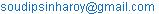Soudip Sinha Roy. A Brief Commentary on Space-Time-Height Relativity. American Journal of Modern Physics. Vol. 5, No. 3, 2016, pp. 39-44.
doi:
10.11648/j.ajmp.20160503.13

Received: May 8, 2016; Accepted: May 19, 2016; Published: May 30, 2016

Abstract: This article contributes a mathematical visualization on the relativity among space-time-height accordingly with the A. Einstein’s special relativity theory. The variation of time with the increment and decrement of space and height is truly focused and elaborated inside. The Lorentz expression about the relativity interprets the relativity of mass correspondingly with the energy of matter. This proposed theory visualize the relativity among three dimensions at a time i.e. space, time and height. Space, time and height are taken as three individual dimensions and all 3D plots are sketched collaborating with these dimensions. To comprehend the theory several explanations are established and several expressions are derived and plotted manually. All the expressions conclude with a great satisfaction that the relativity of time also exists in the collaboration with space and height both instantaneously.

Keywords: Special Relativity, Lorentz Equation, Time-Energy Relativity, Time Dilation

1. Introduction

The relativity principle represents a beautiful correlation among all the living and non-living objects in the scale of velocity, space and time at every instant, on the universe. From the very beginning of its discovery till today, it is still amazing and strongly effective on each and everything that we can see or can’t. The special relativity was firstly introduced by Albert Einstein, by his first paper on relativity in 1905 . After the discovery of that various developing, modification and magnifications have done. As a strict definition "the relativity is the relative interaction of anything with respect to others" , .

Such as the electron moves inside the atom within a proper circumstantial obit, it has always a time, velocity and energy instantaneously , . These all the properties can be said as its self-property of the electron. But when the all characteristics of that electron are measured with respect to another one or more than one electrons then that property is called as relative property. Likely to how much the electron is being effected by others or the current physical characteristics of the electron with respect to another one is said to be as relativity symptoms , .

This paper visualizes a theory of relativity which is based upon the relativity simplification among space, time and height. This theory provides that the relative time variation for two most responsible factors like space and height. This paper contributes how the time changes relatively with the height and space, somehow it runs faster and somehow gets slower down as the responsibility of two causal factors space and height. One special expression is introduced to elaborate the very interesting facts of relativity, which is the modification of Lorentz expression in a different way. This theory spreads a lot and fantastic immunity on this new approach of relativity where the time is literally dependent upon the two factors space & height.

2. Mass Relativity by Lorentz Expression

In classical relativity theory from Einstein’s discovery the time depends upon the speed of the object. The reverse proportionality of time and speed is seriously a fatal evidence for the relativity theory. In this relevant area the Lorentz’s equation collaborate with speed of light and the speed of the object is very useful and salient. Which is given by , ,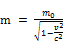(1)

As first introduction with this Lorentz’s equation of the family of relativity theory, its needs to be clarified firstly that v is the speed of object and c is the speed of light according to above eq. 1. In the field of this expression the factor λ =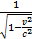is named as Lorentz’s factor. With the Collaboration of factor λ the expression reaches at a relativity premises –.

At the ground of the Lorentz’s expression one drawback is also present. While the speed of the object approaches the speed of the light, then the expression generally approaches the infinity value. In the sense of mass this prediction would be strictly native. As the logical conclusion when the speed of object (v) rise much above the speed of light (c) then the expression experiences imaginary value with itself. This expression also keeps apart the mass from an imaginary value and also it justifies that the speed of any object never ever cross the speed of light [8-10]. In the general theory of relativity if the speed of any particle approaches the speed of light then the mass of the object converts into the energy and perhaps the matter could be invisible. This statement recovers the drawback of the Lorentz’s expression partially, when the eq. 1 approaches an infinite value at a condition of v=c.

3. Demonstration of Space Time Relativity

In the proposed concept the space and the time are strongly interrelated. Variation of any parameter of space and height causes the change in time. Depending upon the Lorentz’s equation one widely concept is presented in this paper. The given expression shows the variation of time which is correlated with height and space. This expression is a moderated form of Lorentz’s equation where the space and the height is installed instead of the velocity of object v and speed of light c respectively [1-5].

In fact for the change of height and space of any object, it is keen difficult to predict the time which changes simultaneously with these two parameters. This time computation is mainly subjected because of successive detection of time of any object located at particular position. How the time gets slower down depending upon d and x parameters and somehow it runs faster, all the things have been clarified by theoretical demonstration. The following expression is a sophisticated approach on the relativity of time which describes all the essential and practical facts on relativity of time. The expression is given as,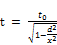(2)

In this expression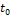is the initial time of the respective object, d and x are denoted as the space and height respectively. This distance is an effective distance between the observer and the particular position of the object. Where t is the relative time of the object which changes with the other parameters. Henceis independent of the variable denominator terms, but t is always dependent on two parameters d & x, those are situated at the denominator place. As apparent as it is to see that if the initial timeis fixed and the distance between the observer and the object increases, as the expression 2, t gets increased. As this case as much the effective distance increases the relative time also increases i.e. likely to the time become faster, as fig. 3. In account of the height of observer x increase with a fixed distance at a particular position then the time gets slower down and x decreases then time increases, as fig. 2.

Case 1: As the fig. 1 (a) shows the space and height is mutually equal. Hence this condition concludes that the time should to have an infinite value as eq. 2, fig. 1 (a). But this proviso is impossible to be explained by this expression.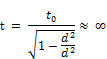Perhaps this condition could not be possible to continue more.

Case 2: In the field of the case 2, the height x is greater than the space d, as fig. 1 (b). As this condition eq. 2 says the time gets collapsed down. This explains that if the height of observer rises much more than the space of the object, then the observer feels a less time cavity, where the time goes slow to slower down, as fig. 1 (b). This situation generates a kind of feelings to the observer that the time of the observer getting gradually slower.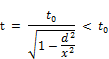Case 3: On the circumstance of case 3, as the space increases much above the value of height, then the time runs faster according to eq. 2. The observer will feel a situation which can be mathematically elaborated as that the time of the observer will be relatively faster, as fig. 1 (c).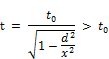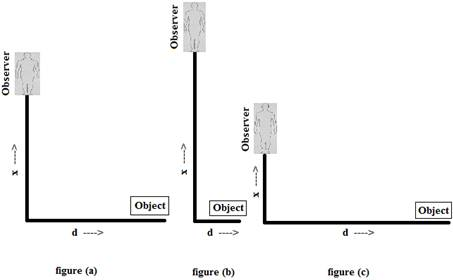Figure 1. Three individual cases of space (d)-height (x).

Limitations of expression 2:

As mentioned above about the drawback previously in the Lorentz’s equation, here this expression also contains similar type of problem. While the space (d) approaches the height (h) then the entire result of the expression tends to infinity . In fact the distance/space always needs to be beyond back of height x. Then only the expression approaches a valid physical significance , . Somehow in case if space crosses the value of height then this expression will have an imaginary impact on itself, which is also insignificant. This imaginary plotting is shown in fig. 4, where all the ambiguity is clarified briefly.

4. Principle of Relativity and Essential Expressions

The principle of relativity requires that the law of the conservation of energy. By means of energy conservation law the author is led to draw the following conclusion on these premises, in conjugation with fundamental equations of mass-energy conservation and energy with frequency of object are , ,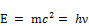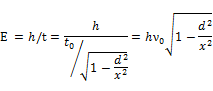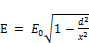(3)

This is an equation which implies the relativity among energy space and height. Literally it is very common to say that the energy of any object (body) always be manipulated by space and height of itself. In one word it is to say the relative energy always depends upon these two fatal parameters.

In the sense of Lorentz’s equation again it is possible to write such , ,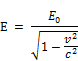That is a very responsible factor for the generation of relative energy of an object. As the speed of the body changes the energy of the body/system changes simultaneously , , , .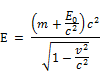In consideration of the expression given above for the kinetic energy of the body, the required energy of the body comes out to be as above equation. As can be said if a body takes up an amount of energy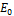, then its inertial mass increases by an amount of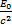.

From the above expression 3, this could be stated that, by means of changing of space and height, the time varies and the energy of the body varies consequently. The author wants to draw a valuable attention on this premises that the energy and the time both are mutually conjugated. One can be influenced by another one at a time of every interaction.

5. Modification of Space Value by Comparing Expression 2 & 3

In section II, as discussed about the space-height relativity the expression 2 is given by,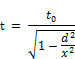If the plank constant h is divided by the above expression then ,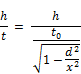A short manipulation says the expression can also be written as follows,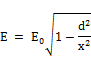The comparison result between eq. 3 and the above derived expression defines,The value of d is manipulated from the above expression to achieve a correlation among speed of light c, speed of object v, and height x. Hence the resultant expression is written as follows ,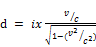(4)

This equation 4, formulates the space, height, speed of light and speed of the object concurrently.

If the value of d is placed in eq. 2, then the expression becomes ,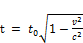(5)

Eq. 5, shows the relativity of time for the influence of speed of light and speed of object , .

Accordance with the decrease of time the energy rises much above its present value. It is apparent to visualize that as the denominator of eq. 2 increases the numerator of eq. 3 also increases. As per the result the time of the body/object falls down and energy increases. On the other hand, the result of increase of time depreciates the energy of the system gradually. This episode explained clearly the relation between time and energy specifically.

6. Graphical Explanations for Proper Relativity Demonstration

Two layers of area are shown in above graph. Where space value is sated to 50 and the height is varied from 1000 to 0. The result of this plot elaborates the time runs faster as the height decreases down , . The effective space or distance between the observer and object is fixed to 50. A slot area of time is shown manually where the relative variation of time is apparently visualized by three coordinates where space, time and height are taken as three different dimensions individually , .

Percentage of incremental time is calculated below,

Hence,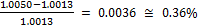Effectively the time gained a factor of 0.36% higher value while the height decreased from 1000 to 500, as fig. 2.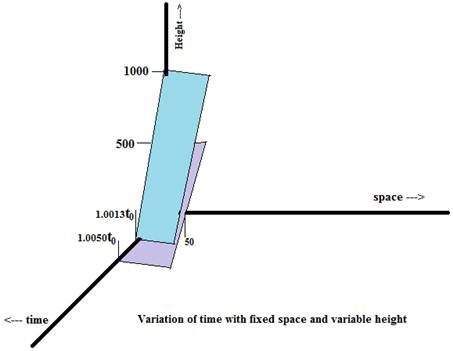Figure 2. The graph is created with fixed space value and variable height.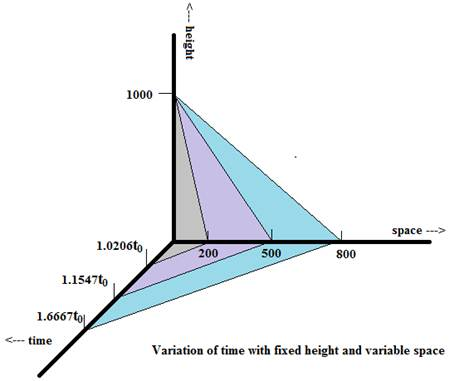Figure 3. This graph is created with a fixed value of height and variable space value.

This plotting explains the variation of time with changing of space while the height is pined to 1000. The above plot clarifies that the time goes faster as the space increases much, but needs to be below the value of the effective height. The colorful segments of the graph shows the accomplishment of different time regions from one point of any variable to another point of the variable. Such as, the gray region shows the time region between the variations of space from 0 to 200. The other colors justify the next space regions accordingly as before one .

The incremental time percentage from one space point to another point is calculated below:

Hence,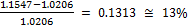This is the effective percentage is 0.13, when the space increases from 200 to 500. So the time also increases by a percentage of 0.13 .

Table 1. Fig. 3, is produced according to the values of table 1.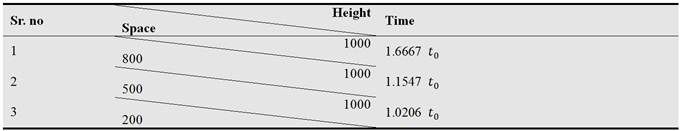In the next case,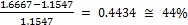Secondly the percentage of increment is 44%, when the space rises up to 800, as fig. 3.

Comparatively it is seen from the results of fig. 2 & fig. 3, that the time rise faster much. Fig. 3 constitutes a relative motion of time where the time runs much faster due to have a change of space . But in fig. 2, the time incrementing phenomena is not that much faster for fixed space value. As said in case 2 in section II, if height rises much over the space, then the time gets collapse down. These two figures 2 & 3 also interpret that the incremental motion of the time is faster than of the fig. 3. Hence this result justifies this statement .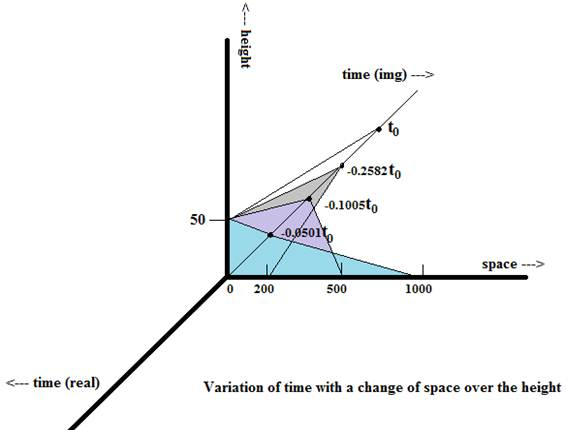Figure 4. This graph is created with fixed height and variable space beyond of height value.

Table 2. Fig. 4, is produced by the table 2.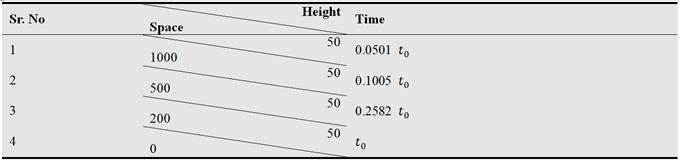Apart from the previous cases, if the space contains a higher value than the height then an imaginary factor arrives and always with a zero real factor . By means of the variation of the space from 0 to 1000 by fixing the height, the resultant phenomenon is elaborated by above graph apparently, as fig. 4. In above figure there have selected two different axis, one is real axis of time and second one the imaginary axis of time. Imaginary part of the axis is displayed just by a thin straight line at opposite of the real axis of the time, as fig. 4.

As all values have been computed by eq. 2, for that reason as initial time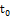is kept constant.

7. Conclusions

Proposed theory is an instance of a special relativity among space-time-height with the collaboration of Einstein’s relativity and Lorentz relativity. In this paper the presented theory imparts an existence of relativity among three responsible factors. The Lorentz’s expression of mass relativity eq. 1, is emulated to catalyze the physical significance of this proposed theory. As the main relativity of time on the response of three factors discussed before, expression 2 is derived from eq. 1. The motivation of the research is clarified by installing three graphical plots corresponding with their respective tables. These plots signify the analogical phenomenon of the time variation criteria. This theory explains the essence of space-time-height relativity and also illustrates the dependency of time on space and height. Author wants to conclude an emerge of a new horizon of special relativity and an accurate relation among three essential factors, by presenting this paper.

Acknowledgements

Author is grateful to the nature to serve the essential actuality to draw up the proper visualization on the special relativity.

References

1. A. Einstein, N. Rosen, "The particle problem in general relativity theory", Institute of Advance Study, Princeton, vol. 48, pp. 73-77, 1st July 1935.
2. A. Einstein, "Foundation of the General Theory Relativity", vol. 6, pp. 146-200, DOC. 30, 1914-1917.
3. Weiskopf, D., Borchers, M., Ertl, T., Falk, M., "Explanatory and illustrative visualization of special and general relativity", IEEE trans. on Visualization and computer graphics, vol. 12, no. 4, p. 522-534, July-Aug. 2006.
4. McCausland, I, "On the special theory of relativity", proc. of the Institution of Electrical Engineers, pp. 1766-1767, Dec. 1972.
5. Sussman, G., Wishdom, J., Farr, W., "Special Relativity", MIT press, pp. 256.
6. Harman, W. W., "Special relativity and the electron", proc. of IRE, vol. 37, no. 11, pp. 1308-1314, Nov. 1949.
7. Tai, C., "Appendix E: Vector analysis in the special relativity", Wiely-IEEE press, Edition 1, pp. 174-180, 1997.
8. Hsiung, P.-K., Thibadeau, R. H., Cox, C.B., Dunn, R.H.P., "Time dilation visualization in relativity", IEEE proc. of Supercomputing ’90, pp. 835-844, 12-16 Nov. 1990.
9. Winkler, G. M. R., "Synchronization and relativity", proc. of IEEE, vol. 79, no. 7, pp. 1029-1039, July 1991.
10. Renshaw, C., "Moving clocks, reference frames and the twin paradox", IEEE trans. on Aerospace and Electronic systems Magazine, vol. 11, no. 1, pp- 27-31, Jan. 1996.
11. Nelson, Robart A., "Relativistic time transfer in Solar system", IEEE international proc. on Frequency control symposium, 2007 joint with the 21th European Frequency and Time Forum, Geneva, pp. 1278-1283.
12. Reed, I. S., "Wave packet with special relativity demonstrating quantum rules, Schrodinger’s equation and propagator integral", IEE proc. on Science, Measurement Technology, vol. 138, no. 4, pp. 223-236, July 1991.
13. Haradhon Kumar Mohajan, "Space-Time Singularities and Raychaudhuri equations", Journal of Natural Science, vol. 1, no. 2, pp. 18-30, Dec. 2013. Available at www.aripd.org/jns.
14. Friedman, M., Serlin, V., Lau, Y. Y., Krall, J., "The physics and applications of modulated intense relativistic electron beams", IEEE proc. of 8th International Conference on High-Power Particle Beams, Novosibirsk, pp. 53-6-, 2-5 July 1990.
15. John Kogut, Washington, D. C., "Introduction to Relativity", ISBN: 978-0-12-417561-7, April 2001. Available at https://www.elsevier.com.
16. G. Kaiser, Lowell, MA, "Quantum physics, Relativity and Complex space-time" ISBN: 978-0-444-88465-7, Dec. 1990. Available at https://www.elsevier.com.
17. Li, T. G. F., et al.: Towards a generic test of the strong field dynamics of general relativity using compact binary coalescence. Phys. Rev. D 85, 082003 (2012).
18. M. D. KRUSKAL'f, "Maximal Extension of Schwarzschild Metric", vol. 119, no. 5, pp. 1743-1745, 1st Sept. 1960. Available at www.springeropen.com.
19. M. Alcubierre, "The Warp Drive: Hyper-Fast Travel within General Relativity," Classical and Quantum Gravity, vol. 11, pp. L73-L77, 1994.
20. T. Ertl, F. Geyer, H. Herold, U. Kraus, R. Niemeier, H.-P. Nollert, A. Rebetzky, H. Ruder, and G. Zeller, "Visualization in Astrophysics," Proc. Eurographics, pp. 149-158, 1989.
21. D. Weiskopf, "Four-Dimensional Non-Linear Ray Tracing as a Visualization Tool for Gravitational Physics," Proc. IEEE Conf. Visualization, pp. 445-448, 2000.
22. D. Ebert and P. Rheingans, "Volume Illustration: Non-Photorealistic Rendering of Volume Models," Proc. EEE Conf. Visualization, pp. 195-202, 2000.
23. Gerald Gwinner, "Experimental tests of time dilation in space relativity", trans. of Worlds Scientific on modern physics letter A, vol. 20, no. 11, pp. 791-805, April 2005.
24. Radwan M. Kassir, "On the relativistic length Contranction and Special Relativity: Twisted conceptios", the general science journal, pp. 1-5, 2015.

BiographySoudip Sinha Roy is a B-TECH student in University of Engineering and Management, Jaipur from 2013. His research area is Quantum physics, Astrophysics, Nanoelectronics. He is a member of the IEEE.
 Contents 1. 2. 3. 4. 5. 6. 7.
Article ToolsAbstractPDF(800K)